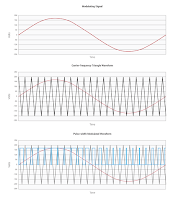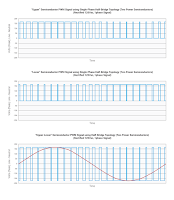## You need to test, we're here to help.

You need to test, we're here to help.

## 05 June 2015

### Testing Challenges in Motor Drive Systems (Part III)Figure 1: An example of PWM for a single power semiconductor
As noted in an earlier post, variable-frequency motor drives (VFDs) display a good amount of variation in terms of architectures and topologies. Another differentiator between VFDs is their application of pulse-width modulation (PWM) techniques.

Voltage output from the VFD is controlled through a variety of modulation schemes, all of which result in simple one-level PWM gate-drive signals (one per power-semiconductor device) and one-level (or, less commonly, more than one level) signals at the output of each phase of the motor drive. When the output voltage is viewed line-line, this one-level signal is a two-level signal. The PWM drive output signals will vary in quality based on the control and modulation technique chosen for the drive.

In general, users refer to these different control techniques as one of many different "sine-modulated" techniques or a "six-step commutation" technique. A sine-modulated technique applies voltage to all three phases simultaneously whereas a six-step commutation technique applies voltage to two of the three windings at any given time.

The one-level PWM gate-drive signals can be determined and generated through a simple carrier-based method or, for sine-modulated techniques, through a more algorithmically complex space-vector (pulse-width) modulation (SVM or SVPWM) method. Carrier-based PWM methods are simpler to program and implement and are lower in cost but have higher harmonic content. SVM methods call for more implementation skill and carry higher costs (due to a faster and more expensive processor in the VFD's embedded control section) but generate lower harmonic content.

### Carrier-Based PWM

In carrier-based PWM schemes, the carrier (high) frequency is most often a triangle wave and the modulating (low-frequency speed control) signal is a sine wave. This approach is often found in simple scalar V/f (sine-modulated) and six-step commutation controls.Figure 2: PWM waveforms for a half-bridge power conversion system
The sinusoidal intersective carrier-based PWM method is the most widely-used approach in new designs. This method uses a constant-amplitude, high-frequency (~1-100 kHz) carrier frequency and a low-frequency control  of ~60 Hz, or the desired VFD output frequency, with a fixed or varying amplitude. The intersection of the modulating sine wave with the carrier frequency creates the width-modulated signal. Take a look at a simple example of  PWM for a single power-semiconducting device in which the PWM signal has a 50% duty cycle at zero volts of the modulating signal (Figure 1).

The carrier frequency can be either a triangle or sawtooth waveform; this defines the intersection with the modulating waveform for purposes of width determination. The example in Figure 1 is for a triangle waveform, but the frequency was less than 1 kHz (i.e., illustrative only) so that the signal could be more easily viewed with a modulating signal of lower frequency.

For full-bridge or half-bridge power-conversion systems, the upper and lower devices are switched independently. Thus, the PWM waveforms for each device are a bit more complicated to "view" and to understand how they relate to the power-conversion device's or drive's output, but the basic concept is the same.Figure 3: PWM waveforms for a full-bridge power conversion system
For example, in a half-bridge configuration, there is an upper and lower device; both switch in a complementary fashion to create the full output waveform. In Figure 2, the upper device's PWM waveform is at top, the lower device's PWM waveform is at center, and the total output (upper minus lower) PWM waveform is at bottom (the modulating and carrier-frequency waveforms are omitted in this example but they are the same as in Figure 1).

In a full-bridge, or H-bridge, design, the upper and lower waveforms and output PWM waveforms appear as shown in Figure 3. For a three-phase design, one modulating signal is created in the microcontroller and then phase-shifted by 120° or 240° to create the other two modulating signals for the other two phases.

Note that both series power-semiconductor devices in a half-bridge, full-bridge, or cascaded H-bridge topology cannot be switching on at the same time, which may result in "shoot-through" and device failure. Thus, some minimum dead time before and after switching is built into the controls to avoid having both devices switched on simultaneously.

### Space Vector (Pulse-Width) Modulation (SVM or SVPWM)

In SVM, a matrix transformation is used to transform a three-phase voltage signal that corresponds to the rotating stator magnetic field into a single rotating "space vector" in a two-dimensional reference frame. This simplifies calculation of a single voltage magnitude and angle, which is then inverse-transformed back to a three-phase voltage signal from which the gate drive PWM signals can be generated.

SVM is a more algorithmically complex modulation scheme compared to a conventional carrier-based PWM, but it allows suppression of certain harmonics for an overall improvement of the VFD's harmonic performance. There are several variants of space-vector modulation based on the alignment of the pulse widths within a defined "time slot." But in the final analysis, the VFD's PWM output signals look essentially the same as with carrier-based PWM schemes.